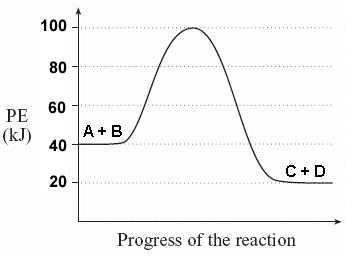Problem: For the following reaction, answer the following questions:a. Estimate the activation energy (provide both value and unit)b. Does the graph represent an endothermic or exothermic reaction?c. Determine the enthalpy (ΔH) for the reaction product.

FREE Expert Solution
99% (118 ratings)
Problem Details

For the following reaction, answer the following questions:

a. Estimate the activation energy (provide both value and unit)

b. Does the graph represent an endothermic or exothermic reaction?

c. Determine the enthalpy (ΔH) for the reaction product.What scientific concept do you need to know in order to solve this problem?

Our tutors have indicated that to solve this problem you will need to apply the Enthalpy concept. You can view video lessons to learn Enthalpy Or if you need more Enthalpy practice, you can also practice Enthalpy practice problems .

What is the difficulty of this problem?

Our tutors rated the difficulty of For the following reaction, answer the following questions:a... as medium difficulty.

How long does this problem take to solve?

Our expert Organic tutor, Jonathan took 1 minute to solve this problem. You can follow their steps in the video explanation above.

What professor is this problem relevant for?

Based on our data, we think this problem is relevant for Professor Henricks' class at KSU.